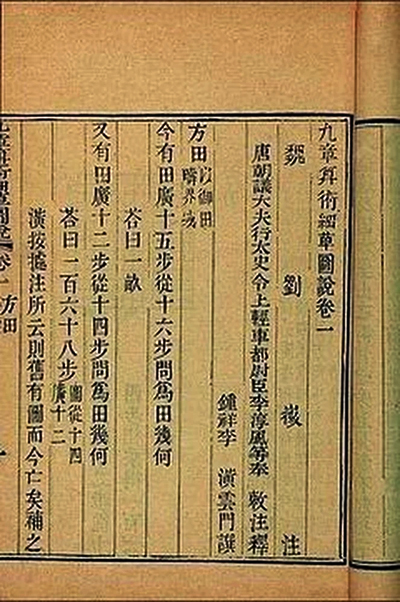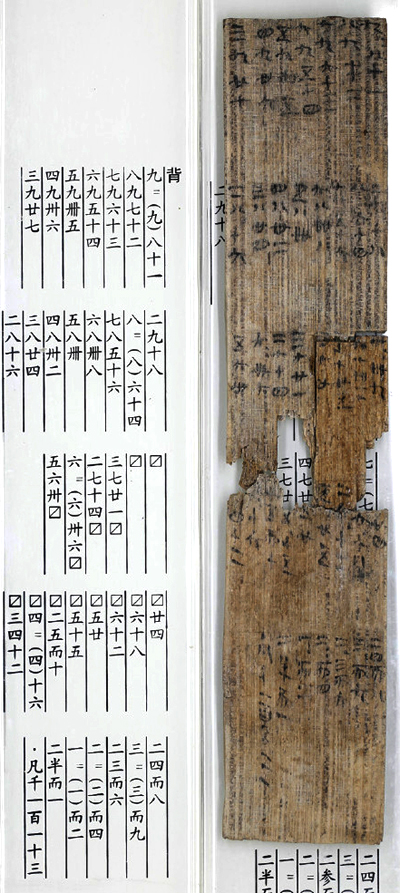#Nine Chapters Math is the most important math book in Chinese history prior to the 20th century.

Most researchers believe ancient mathematics in China and in the Mediterranean worked independently up to the time when the Nine Chapters Math reached its final form that was around the first century.

“The method of chapter 7 was not found in Europe until the 13th century, and the method of chapter 8 uses Gaussian elimination before Carl Friedrich Gauss (1777–1855) … There is also the mathematical proof given in the treatise for the Pythagorean theorem.” (The Nine Chapters on the Mathematical Art – Wikipedia)

# Nine Chapters in the Book:

1) Rectangular Fields (方田): Calculation of areas of fields in various shapes; manipulation of vulgar fractions;

2) Millet and Rice (粟米): Rates and prices conversion between different commodities;

3) Proportional Distribution (衰分): Promotional rates for distribution of commodities and currency;

4) The Lesser Breadth (少广): Division by mixed numbers; extraction of square and cube roots; dimensions, area and volume of circle and sphere;

5) Consultations on Works (商功): Volumes of solids in various shapes;

6) Equitable Taxation (均輸): Solutions to more advanced problems on proportion;

7) Excess and Deficit (盈不足): Linear problems solved using the concept of false position;

8) The Rectangular Array (方程): Systems of linear equations solved by a concept similar to Gaussian elimination.

9) Base and Altitude (勾股): Solutions to problems known in the West as the Pythagorean theorem.

More details on the topic at
The Nine Chapters on the Mathematical Art (wikipedia)

# Nine Times Tables

In a rescue dig of a Qin Dynasty relics in 2002 in Shaanxi, Nine Times Table written on Bamboo slips were unearthed.Right: the unearthed math chat on bamboo slips;
Left: a printed clearer version of the math chat.

This 2,200-year-old Nine Times Tables is said to be almost identical to the one studied by Chinese kids in school today, except the ancient version also covers some fractional operations.